# Bridging the Gap- Modeling and Methods in Maths(Part 1)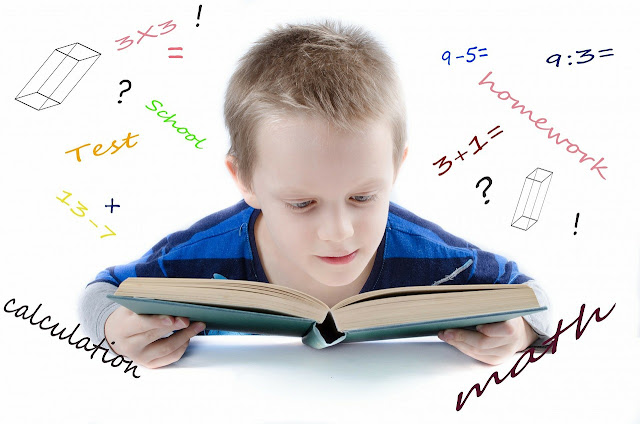Learning Maths

Maths is a subject which requires a lot of practice in order to be familiar with it. There are many different concepts which one need to master and formulas to memorise in order to complete the array of questions within the given time.

In the last post I gave an overview of the components tested in a Math’s paper.  In this post I will be showing how Long Answer Questions can be solved using different methods.

**These are actual questions which are taken from the homework worksheets that Monkey boy has brought  home and  I have worked through together with him.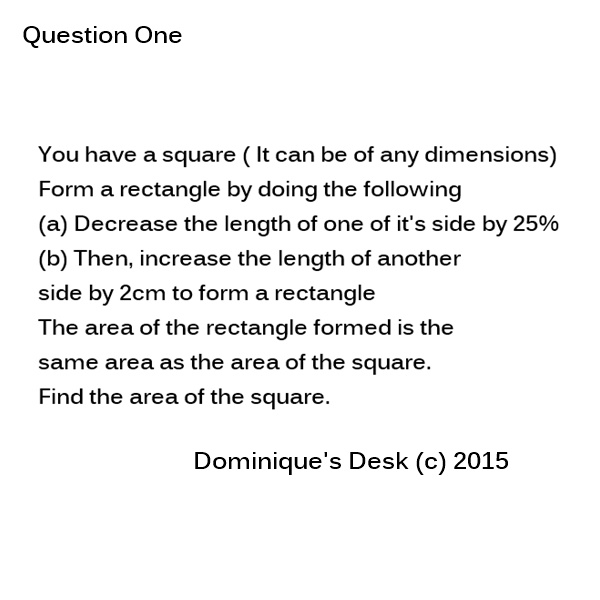Maths Question

My first inclination was to see if it was possible to draw a model to analyse the question.  After pondering on it for a while both of us found it more complicated to explain if we drew a model for it and eliminated it from the ” potential way to solve the question” list.

We decided to use the Guess/List and check method as there were two different unknowns which had to be identified.Square to rectangle

We worked through the possible  chance of  what the two different lengths of the rectangle could be given ( X and Y) given that the square was of a certain length for each side.

 Length of Side Area X (at 75%) Y at ( +2) Area of Rectangle 10 100cm² 10-2 = 8 10 + 2 = 12 8 x 12 = 94 cm² 8 64cm² 8- 2 = 6 8 +3 = 10 6 x 10= 60 cm² 6 36cm² 6-2.5=4.5 6=2= 8 4.5 x 8 = 36cm²

As shown above I assumed that each side of the square was 10cm so the area of the square would be 100 cm2.

Using the projections that ” one side had to be decreased by 25%”  the possibility of 1 side of the rectangle would  be 10-2 = 8 cm.

Following through on the part ” increase the length of the other side by 2cm” I added 2 to 10 to get 12cm.  However when you multiplied both sides to find the area of the rectangle I got 8 x 12 = 94 cm2.

This is not correct as there has been a change in the total area from 100cm2.    to  94 cm2.

I slowly moved down the list of possible numbers till I got to 6 cm as the length of the side of the square  and that the area of the square was equal to the area of the rectangle.

That was how we found the answer for this particular question.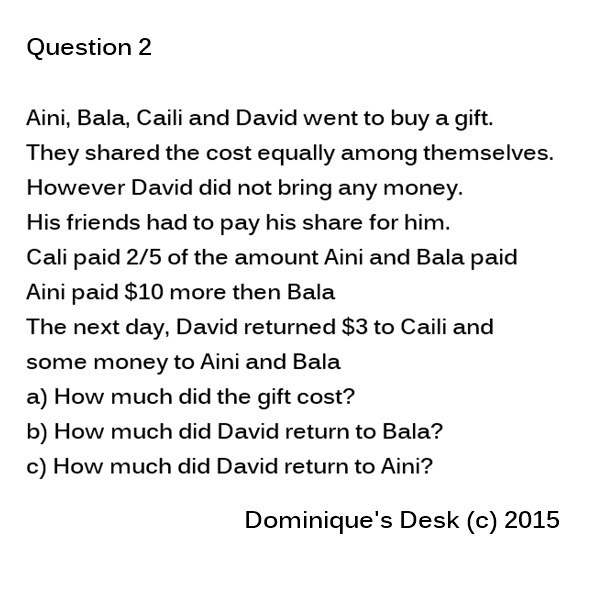If you are anything like me you too may draw a blank after reading through the whole question. I had to re-read through it a couple of times just to process what the questions was asking.  I found the question really complicated.

I seriously don’t remember having to attempt such complex questions when I was in Primary 5 back then. The difficultly level of questions attempted in Maths has certainly increased a lot!!

To solve this sum we had to use the Working backward method.

We start off from the sentence

” David paid \$3 back to Cali”

If we divide the amount David should have paid in 2 parts

The amount Cali paid : The amount  Aini and Bala paid = 2u: 5u

Therefore if all the kids paid  and equal proportion of the present  each

2 u (x4) : 5 u (x4)

8 u : 20 u

Cost of the present = 28u

Each child would have to pay 7 u. However Calia paid  1u more as she paid part of David share so

8u- 7u = 1 u

1 u = \$3

28 u = \$3 x 28

= \$84

a) The price of the present is \$84

The amount of money Aini and Bala paid in total = 20 u (\$60)

Based on the fact that Aini paid \$10 more then Bala we find the amount the Aini paid by doing the following.  (60 + 10) / 2 = \$35

Bala pays \$10 lesser

\$35 – \$10 = \$25

(Checking – \$25 + \$35 = \$60)

Since each person originally needed to pay 7 u = \$21

David had to return \$25-\$21 = \$4 to Bala  and \$35-\$21 = \$14 to Aini

b) David returns \$4 to Bala

c) David returns \$14 to Aini.

In the next post I will go through the remaining two methods- Modelling and Looking at a pattern to show how they can be applied in problem solving.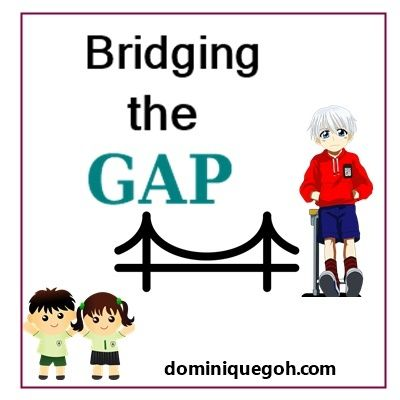Post in this series

9) Bridging the Gap- Upper and Lower Primary Science

10) Bridging the Gap-  Moving from Lower to Upper Primary

11) Bridging the Gap- Moving from Lower to Upper Primary ( Part 2)

# Identifying the Gap between P4 and P5 Maths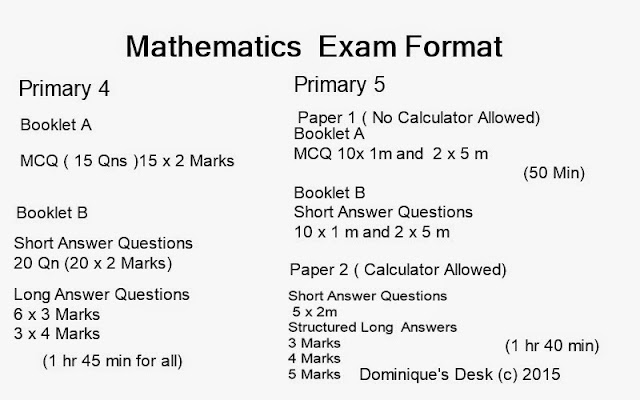The mathematics exam format

Mathematics has always been one of the subjects which the Monkey boy does not have major difficulties in. He has  always been strong in this subject and achieves  between 80-90 for most of his Math’s papers.

However as his move from one level to another there is in an increase in the depth of each topic which he would have to master and maintaining good grades gets harder.

As you can see from the table above there are now two papers that have to be completed for the Maths Exam instead of one.

The time for the exam has been extended from 1hr 45 min to  50 min + 1h 40min. Within the extra 45 min 2 Papers have to be completed instead of just one.

Even though the calculator is allowed to be used for  Paper 2 the questions that are posed are much more complicated and require multiple steps to solve. ( On average 4-6 steps per question)

In  a  Primary 4 Maths exam the student has to complete  44 questions  while in Primary 5 they need to complete 48 questions within an additional 45 minutes.

Even though there are only 4 more questions it can pose much difficult to the student.  These additional questions are within the  Structured Long Questions in Paper 2 which adds up to a   50% weightage of the whole paper.

This certainly discounts the fact that even though the  calculator is allowed for use in Paper 2 it doesn’t mean the questions are easier to complete.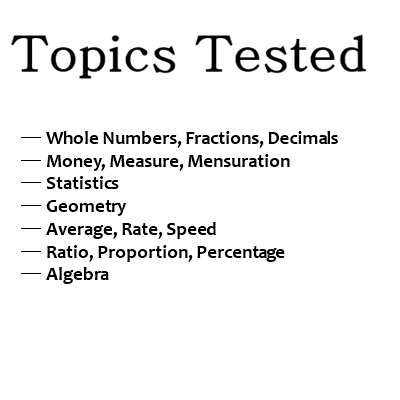Maths Topics tested

Most of the topics that are tested are built up on concepts that have been introduced since Primary 1.  From the emphasis on the  four operations ( + – X and  ÷) to   various skills and processes which are  required to solve the problem a vast array of problem solving techniques need to be implemented.

## Methods used to solve  Long Answer questions

There are several different methods which can be used to solve Long Answer questions.  The most common method of solving structured long answers is using the Model method.

This method is promoted in solving long answer questions as pupils can represent the mathematical relationships in a problem pictorially. This helps them understand the problem and plan steps for the solution. The pictorial form also helps pupils visualise what could otherwise be abstract concepts. (Fractions, Ratio & Percentage)

There are other methods which can be used to solve the Long Answer question when using the Model method doesn’t seem relevant to the posed question.

The other methods that are used are

– List and Check

– Looking for a pattern

– Working backwards.

I personally think it is important for the student to know how to use the various different methods that they have been taught in school.

In the next post I will be sharing with you how I  help Monkey boy reinforce the concepts he has learned in Maths.Post in this series

9) Bridging the Gap- Upper and Lower Primary Science

10) Bridging the Gap-  Moving from Lower to Upper Primary

11) Bridging the Gap- Moving from Lower to Upper Primary ( Part 2)